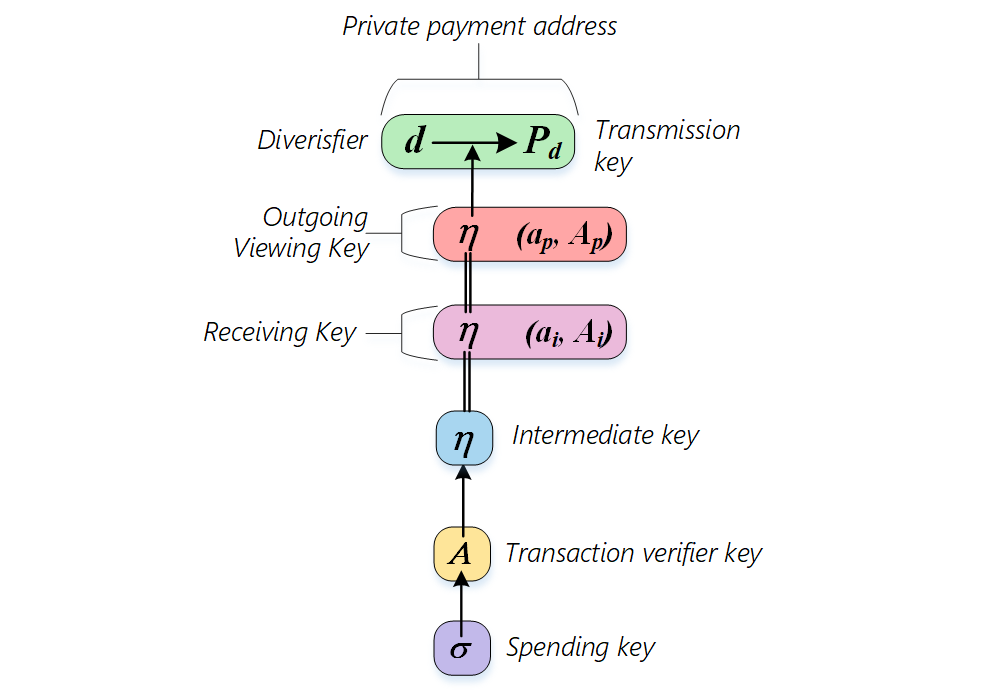# ZeroPool Keys

ZeroPool is based on complex cryptography. There are several keys needed for private transactions. The relationship between these keys is presented in the scheme below.• Spending key ($\sigma$) is the top secret key. It is used to derive other keys and to sign transactions. At a high level it is just a random 256-bit number which should be stored securely on the client side. The simplest way to get a spending key is to produce a random number. But in a production client software implementation more complex approaches should be used (e.g. hierarchical deterministic wallets). Concrete approaches for spending key derivation by a client are not discussed here.
• Transaction verifier key ($A$) is used for transaction signature verification. It's derived from the spending key and multiplied by the generator point in the JubJub elliptic curve field: $A = \sigma G$
• Intermediate key ($\eta$) is derived from the $A$ key by the $Poseidon$ hash function:$\eta = Hash(A.x)$. It is used in several cases:
• to calculate the account nullifier
• to obtain the account balance and transaction history
• to decrypt incoming notes
• Receiving key is used to decrypt incoming notes in the memo block. It is a combination of the intermediate key and ephemeral key generated for each note.
• Outgoing viewing key is used to decrypt the whole memo block in the transaction which is initiated by itself.
• Private payment address $(d, P_d)$ - is a set of random diversifiers $d$ and point $P_d = \eta G_p = \eta \text{ToSubGroupHash}_{E(F_r)}(d)$